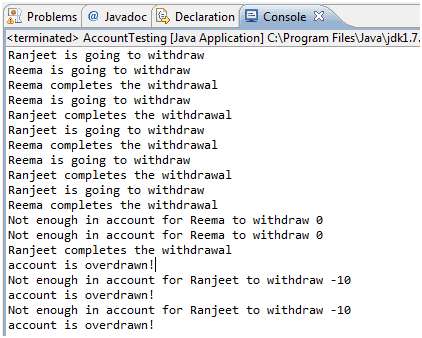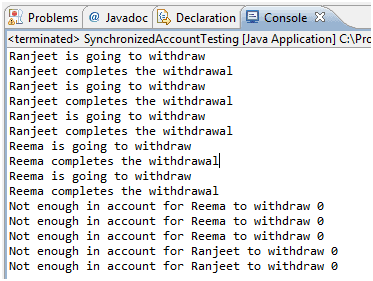# Java Code Synchronization

## Introduction

During the design stage of a multi-threaded application’s development, you should consider the possibility of a so-called race condition, which happens when multiple threads need to modify the same program resource at the same time (concurrently). The classic example is when a husband and wife are trying to withdraw cash from different ATMs at the same time.

In below example, we have a class called Account that represents a bank account. To keep the code short, this account starts with a balance of 50 and can be used only for withdrawals. The withdrawal will be accepted even if there isn't enough money in the account to cover it. The account simply reduces the balance by the amount you want to withdraw:

Java Code:

``````package synchronization;
public class Account {
private int balance = 50;
public int getBalance() {
return balance;
}
public void withdraw(int amount) {
balance = balance - amount;
}
}
```
```

Imagine a couple, Ranjeet and Reema, who both have access to the account and want to make withdrawals. But they don't want the account to ever be overdrawn. Below AccountTesting.java class will start two threads and both thread trying to withdraw money from same account object in the loop. Withdrawal is two steps process :

1. Check the balance.

2. If there's enough in the account (withdraw10), make the withdrawal.

Java Code:Go to the editor

``````public class AccountTesting implements Runnable {
private Account acct = new Account();
public static void main(String[] args) {
AccountTesting r = new AccountTesting();
one.setName("Ranjeet");
two.setName("Reema");
one.start();
two.start();
}
@Override
public void run() {
for (int x = 0; x < 5; x++) {
makeWithdrawal(10);
if (acct.getBalance() < 0) {
System.out.println("account is overdrawn!");
}
}
}
private void makeWithdrawal(int amt) {
if (acct.getBalance() >= amt) {
try {
} catch (InterruptedException ex) {
}
acct.withdraw(amt);
} else {
System.out.println("Not enough in account for " + Thread.currentThread().getName() + " to withdraw " + acct.getBalance());
}
}
}

class Account {
private int balance = 50;
public int getBalance() {
return balance;
}
public void withdraw(int amount) {
balance = balance - amount;
}
}
```
```

Output:Although each time you run this code the output might be a little different, let's walk through this particular example using the numbered lines of output. For the first four attempts, everything is fine.This problem is known as a "race condition," where multiple threads can access the same resource (typically an object's instance variables) and can produce corrupted data if one thread "races in" too quickly before an operation that should be "atomic" has completed.

Synchronization

Synchronization is the solution for this problem. A special keyword, synchronized, prevents race conditions from happening. This keyword places a lock (a monitor) on an important object or piece of code to make sure that only one thread at a time will have access.

How do you protect the data? You must do two things:

• Mark the variables private.
• Synchronize the code that modifies the variables.

We can solve all of Ranjeet and Reema's problems by adding one word to the code. We mark the makeWithdrawal() method synchronized as follows:

here is the modified Java Code

``````public class SynchronizedAccountTesting implements Runnable {
private Account acct = new Account();
public static void main(String[] args) {
SynchronizedAccountTesting r = new SynchronizedAccountTesting();
one.setName("Ranjeet");
two.setName("Reema");
one.start();
two.start();
}
@Override
public void run() {
for (int x = 0; x < 5; x++) {
makeWithdrawal(10);
if (acct.getBalance() < 0) {
System.out.println("account is overdrawn!");
}
}
}
private synchronized void makeWithdrawal(int amt) {
if (acct.getBalance() >= amt) {
try {
} catch (InterruptedException ex) {
}
acct.withdraw(amt);
} else {
System.out.println("Not enough in account for " + Thread.currentThread().getName() + " to withdraw " + acct.getBalance());
}
}
}
class Account {
private int balance = 50;
public int getBalance() {
return balance;
}
public void withdraw(int amount) {
balance = balance - amount;
}
}
```
```

Output:Locks the entire method withdrawCash()so no other thread will get access to the specified portion of code until the current (locking) thread has finished its execution of withdrawCash().The locks should be placed for the shortest possible time to avoid slowing down the program: That’s why synchronizing short blocks of code is preferable to synchronizing whole methods.

Every object in Java has a built-in lock that only comes into play when the object has synchronized method code. When we enter a synchronized non-static method, we automatically acquire the lock associated with the current instance of the class whose code we're executing.

Summary:

• The synchronized methods prevent more than one thread from accessing an object's critical method code simultaneously.
• You can use the synchronized keyword as a method modifier, or to start a synchronized block of code.
• To synchronize a block of code (in other words, a scope smaller than the whole method), you must specify an argument that is the object whose lock you want to synchronize on.
• While only one thread can be accessing synchronized code of a particular instance, multiple threads can still access the same object's unsynchronized code.

Java Code Editor: